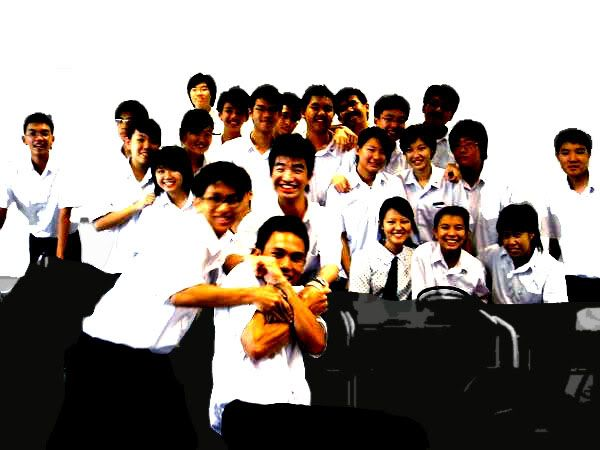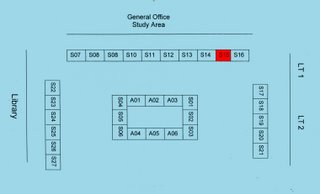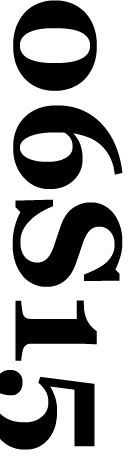Tuesday, April 25, 2006GREEN BAZAAR

Here are the details. (:

Date: 26th April 2006 (Wednesday)
Time: 9 am - 3 pm
Venue: Concourse

Booth Location:
1. One table & two chairs will be allocated to the class at the concourse area.
2. We are allowed to start decorating and advertising on 24th.
3. Location of stall is shown in the picture above.

Manpower at each booth:
1. Each stall is allowed to have one or two students manning the booth at all times (9am-3pm). We can set up their stalls from 9am onwards.
2. Each student should not miss more than 2 periods of lessons.

Duty roster:
0900-0930; BoonKiat &Ernah
0930-1000; Eudora &GuanHong
1000-1030; ChyeTee &Jessica
1030-1100; ChunYu &Jonathan
1100-1130; MingFen &Lionel
1130-1200; Candy &Christine
1200-1230; Justin &TianHao
1230-1300; Alvin &JingXiang
1300-1330; TunKuang &Janson
1330-1400; Andy &EngKien
1400-1430; HuiMing &Michelle
1430-1500; SiewTing &Gabriel

:D 2:05 AM

HOUSE/PE SHIRTS &SHORTS.

Hello. (:

The following will be written in this order:
- Name, House
- No. of house shirts = Amt to be paid.
- No. of PE shirts = Amt to be paid.
- No. of PE shorts = Amt to be paid.
- Total Amt.

Please double check. If there're any errors, pls msg me. AND, everyone MUST bring the money tmr, or else the entire class would not be able to collect their shirts.NOTE: The \$2 included in the total amt is not gst for Green Bazaar.

* * *

1. Michelle, Darwin
1 x \$8.50 = \$8.50
0 x \$7.50 = \$0
1 x \$7.00 = \$7.00
Total Amt = \$8.50 + \$0 + \$7.00 + \$2.00 = \$17.50

2. SiewTing, Keller
1 x \$8.50 = \$8.50
0 x \$7.50 = \$0
0 x \$7.00 = \$0
Total Amt = \$8.50 + \$0 + \$0 + \$2.00 = \$10.50

3. HuiMing, Owens
1 x \$8.50 = \$8.50
0 x \$7.50 = \$0
1 x \$7.00 = \$7.00
Total Amt = \$8.50 + \$0 + \$7.00 + \$2.00 = \$17.50

4. Mingfen, Darwin
2 x \$8.50 = \$17.00
1 x \$7.50 = \$7.50 2 x \$7.00 = \$14.00
Total Amt = \$17.00 + \$7.50 + \$14.00 + \$2.00 = \$40.50

5. Christine, Polo
1 x \$8.50 = \$8.50
1 x \$7.50 = \$7.50
1 x \$7.00 = \$7.00
Total Amt = \$8.50 + \$7.50 + \$7.00 + \$2.00 = \$25.00

6. Ernah, Owens
1 x \$8.50 = \$8.50
0 x \$7.50 = \$0
0 x \$7.00 = \$0
Total Amt = \$8.50 + \$0 + \$0 + \$2.00 = \$10.50

7. Eudora, Keller
1 x \$8.50 = \$8.50
0 x \$7.50 = \$0
0 x \$7.00 = \$0
Total Amt = \$8.50 + \$0 + \$0 + \$2.00 = \$10.50

8. Chye Tee, Owens
1 x \$8.50 = \$8.50
1 x \$7.50 = \$7.50
2 x \$7.00 = \$14.00
Total Amt = \$8.50 + \$7.50 + \$14.00 + \$2.00 = \$32.00

9. Candy, Darwin
1 x \$8.50 = \$8.50
0 x \$7.50 = \$0
0 x \$7.00 = \$0
Total Amt = \$8.50 + \$0 + \$0 + \$2.00 = \$10.50

10. Jessica, Owens
1 x \$8.50 = \$8.50
0 x \$7.50 = \$0
0 x \$7.00 = \$0
Total Amt = \$8.50 + \$0 + \$0 + \$2.00 = \$10.50

11. TianHao, Darwin
1 x \$8.50 = \$8.50
1 x \$7.50 = \$7.50
2 x \$7.00 = \$14.00
Total Amt = \$8.50 + \$7.50 + \$14.00 + \$2.00 = \$32.00

12. Andy, Keller
2 x \$8.50 = \$17.00
0 x \$7.50 = \$0
2 x \$7.00 = \$14.00
Total Amt = \$17.00 + \$0 + \$14.00 + \$2.00 = \$33.00

13. TunKuang, Polo
1 x \$8.50 = \$8.50
0 x \$7.50 = \$0
1 x \$7.00 = \$7.00
Total Amt = \$8.50 + \$0 + \$7.00 + \$2.00 = \$17.50

14. Jonathan, Bryon
1 x \$8.50 = \$8.50
0 x \$7.50 = \$0
2 x \$14.00 = \$14.00
Total Amt = \$8.50 + \$0 + \$14.00 + \$2.00 = \$24.50

15. Justin, Nobel
1 x \$8.50 = \$8.50
0 x \$7.50 = \$0
0 x \$7.00 = \$0
Total Amt = \$8.50 + \$0 + \$0 + \$2.00 = \$10.50

16. EngKien, Owens
2 x \$8.50 = \$17.00
0 x \$7.50 = \$0
2 x \$7.00 = \$14.00
Total Amt = \$8.50 + \$0 + \$14.00 + \$2.00 = \$24.50

17. Gabriel, Byron
1 x \$8.50 = \$8.50
0 x \$7.50 = \$0
1 x \$7.00 = \$7.00
Total Amt = \$8.50 + \$0 + \$7.00 + \$2.00 = \$17.50

18. Lionel, Darwin
1 x \$8.50 = \$8.50
0 x \$7.50 = \$0
0 x \$7.00 = \$0
Total Amt = \$8.50 + \$0 + \$0 + \$2.00 = \$10.50

19. Alvin, Darwin
1 x \$8.50 = \$8.50
0 x \$7.50 = \$0
0 x \$7.00 = \$0
Total Amt = \$8.50 + \$0 + \$0 + \$2.00 = \$10.50

21. ChunYu, Owens
2 x \$8.50 = \$17.00
2 x \$7.50 = \$15.00
3 x \$7.00 = \$21.00
Total Amt = \$17.00 + \$15.00 + \$21.00 + \$2.00 = \$55.00

22. GuanHong, Polo
1 x \$8.50 = \$8.50
0 x \$7.50 = \$0
2 x \$7.00 = \$14.00
Total Amt = \$8.50 + \$0 + \$14.00 + \$2.00 = \$24.50

23. Janson, Owens
1 x \$8.50 = \$8.50
0 x \$7.50 = \$0
1 x \$7.00 = \$7.00
Total Amt = \$8.50 + \$0 + \$7.00 + \$2.00 = \$17.50

24. BoonKiat, Owens
1 x \$8.50 = \$8.50
0 x \$7.50 = \$0
0 x \$7.00 = \$0
Total Amt = \$8.50 + \$0 + \$0 + \$2.00 = \$10.50

25. JingXiang, Polo
1 x \$8.50 = \$8.50
0 x \$7.50 = \$0
0 x \$7.00 = \$0
Total Amt = \$8.50 + \$0 + \$0 + \$2.00 = \$10.50

Thanks!

:D 1:22 AM!OH6S15

Classparent ;MDM LIM
TWENTYFOUR souls
PIONEERjc

!SHOUTS

!ARCHIVES
• April 2006
• May 2006
• June 2006
• July 2006
• August 2006